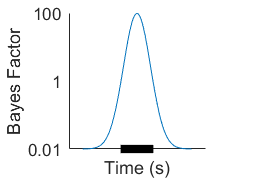#### Howdy, Stranger!

It looks like you're new here. If you want to get involved, click one of these buttons!

Supported by

# Sample by sample time series analysis with generalTestBF

edited February 2017

Hi all,

I have a question about the use of generalTestBF from the BayesFactor package for R. I believe I followed all the steps provided on http://bayesfactorpcl.r-forge.r-project.org/#generalTestBF correctly, but I am not entirely sure if I am interpreting the results correctly.

For the analysis of the time series of a continuous dependent variable (pupil size), I have constructed general linear mixed models (GLMM) using generalTestBF for each sample in the time series. In the GLMM, the dependent variable is modeled as a function of two 2-level factors with a random intercept for each subject

``````m <- generalTestBF(
pupil ~ task * luminance + subject,
data = tmpdata,
whichRandom = 'subject',
whichModels = 'all',
iterations = 10000,
neverExclude = 'subject'
)
``````

To find evidence for an effect of any of the independent variable (IV) I summed the Bayes factors of all the models containing that IV, and divided that by the sum of Bayes factors of all the models that did not contain that IV.

After running the above model for all samples in the time series, you end up with, what I interpreted as, a Bayes Factor time series. Example plot below.To interpret this Bayes factor time series, I picked the commonly used BF > 3 to indicate epochs with evidence in favor of an effect of the IV on the dependent variable (in the example plot illustrated by the black horizontal bar).

Is my interpretation of this output correct? Or am I missing an important step in the analysis?

Jasper